# Boxplots in R Language

A box graph is a chart that is used to display information in the form of distribution by drawing boxplots for each of them. This distribution of data based on five sets (minimum, first quartile, median, third quartile, maximum).

Boxplots are created in R by using the boxplot() function.

Syntax:

`boxplot(x, data, notch, varwidth, names, main)`

Parameters:

• x: This parameter sets as a vector or a formula.
• data: This parameter sets the data frame.
• notch: This parameter is the label for horizontal axis.
• varwidth: This parameter is a logical value. Set as true to draw width of the box proportionate to the sample size.
• main: This parameter is the title of the chart.
• names: This parameter are the group labels that will be showed under each boxplot.

#### Creating a Simple Box Plot

Approach: To understand how we can create a boxplot:

1. We use the data set “mtcars”.
2. Let’s look at the columns “mpg” and “cyl” in mtcars.

Example:

 `input` `<``-` `mtcars[, c(``'mpg'``, ``'cyl'``)] ` `print``(head(``input``)) `

Output: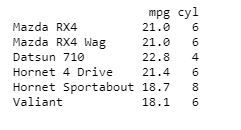#### Creating the Boxplot

Approach: Creating the Boxplot graph:

1. Take the parameters which are required to make boxplot.
2. Now we draw a graph for the relation between “mpg” and “cyl”.

Example:

 `# Plot the chart. ` `boxplot(mpg ~ cyl, data ``=` `mtcars,  ` `        ``xlab ``=` `"Number of Cylinders"``, ` `        ``ylab ``=` `"Miles Per Gallon"``,  ` `        ``main ``=` `"Mileage Data"``) `

Output: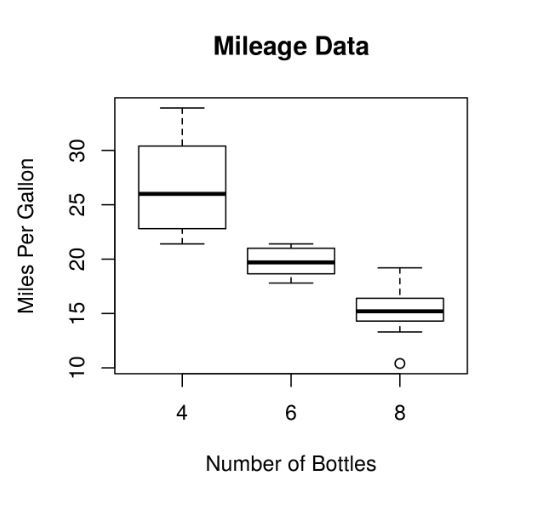#### Boxplot using notch

Approach: To draw a boxplot using a notch:

1. With the help of notch, we can find out how the medians of different data groups match with each other.
2. We are using xlab as “Quantity of Cylinders” and ylab as “Miles Per Gallon”.

Example:

 `# Plot the chart. ` `boxplot(mpg ~ cyl, data ``=` `mtcars,  ` `        ``xlab ``=` `"Number of Cylinders"``, ` `        ``ylab ``=` `"Miles Per Gallon"``,  ` `        ``main ``=` `"Mileage Data"``, ` `        ``notch ``=` `TRUE,  ` `        ``varwidth ``=` `TRUE,  ` `        ``col ``=` `c(``"green"``, ``"red"``, ``"blue"``), ` `        ``names ``=` `c(``"High"``, ``"Medium"``, ``"Low"``) ` `        ``) `

Output: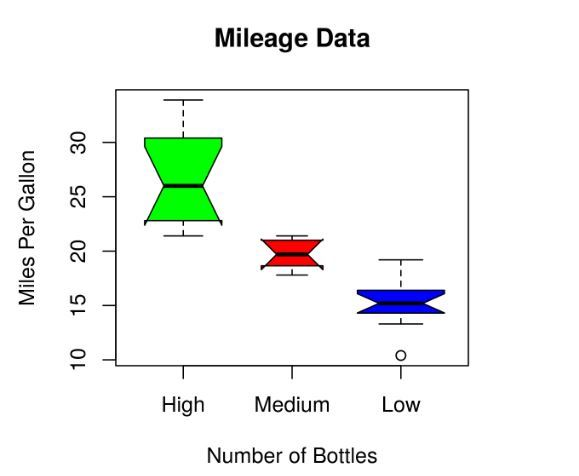#### Creating a Violin Plots

Approach: To understand how we can create a boxplot:

2. The violin plots are created with the help of vioplot() function.

Example:

 `# Loading the vioplot package    ` `library(vioplot)   ` `    `  `# Creating data for vioplot function   ` `x1 <``-` `mtcars\$mpg[mtcars\$cyl ``=``=` `4``]   ` `x2 <``-` `mtcars\$mpg[mtcars\$cyl ``=``=` `6``]   ` `x3 <``-` `mtcars\$mpg[mtcars\$cyl ``=``=` `8``]   ` ` `  `# Creating vioplot function   ` `vioplot(x1, x2, x3,  ` `        ``names ``=` `c(``"4 cyl"``, ``"6 cyl"``, ``"8 cyl"``),   ` `        ``col ``=``"green"``)   ` ` `  `# Setting title    ` `title(``"Violin plot example"``)  `

Output: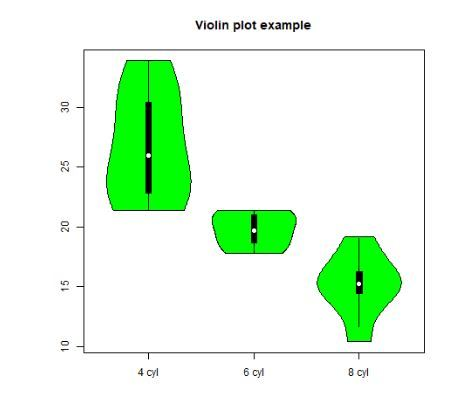#### Two-Dimensional Boxplot Extension

Approach: To understand how we can create a dimensional boxplot, need to do:

1. Creating bagplot function to explain it in an easy way.
2. In bagplot, taking xlab=”Car Weight” in X-axis, ylab=”Miles Per Gallon” in Y-axis .

Example:

 `# Loading aplpack package   ` `library(aplpack)   ` `    `  `# Creating bagplot function   ` `attach(mtcars)   ` `bagplot(wt, mpg, xlab ``=``"Car Weight"``,  ` `           ``ylab ``=``"Miles Per Gallon"``,   ` `       ``main ``=``"2D Boxplot Extension"``)  `

Output: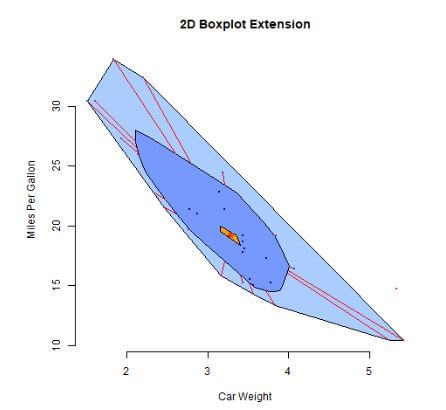My Personal Notes arrow_drop_upIf you like GeeksforGeeks and would like to contribute, you can also write an article using contribute.geeksforgeeks.org or mail your article to contribute@geeksforgeeks.org. See your article appearing on the GeeksforGeeks main page and help other Geeks.

Please Improve this article if you find anything incorrect by clicking on the "Improve Article" button below.

Improved By : Akanksha_Rai

Article Tags :

1

Please write to us at contribute@geeksforgeeks.org to report any issue with the above content.